A compound A of formula C3H6Cl2 on reaction with alkali can give B of formula C3H6O or C of formula C3H4. B on oxidation gave a compound of the formula C3H6O. C with dilute H2SO4 containing Hg2+ ion gave D of formula C3H6O, which with bromine and NaOH gave the sodium salt of C2H4O2. Then A is :

(a) CH3CH2CHCl2

(b) CH3CCl2CH3

(c) CH2ClCH2CH2Cl

(d) CH3CHClCH2Cl

Complete Question Bank + Test Series
Complete Question Bank

Difficulty Level:

Identify the product (A) in following reaction series, ${\mathrm{CH}}_{3}\mathrm{CN}\stackrel{\mathrm{Na}/{\mathrm{C}}_{2}{\mathrm{H}}_{5}\mathrm{OH}}{\to }$(X)$\stackrel{{\mathrm{HNO}}_{2}}{\to }$(Y)$\stackrel{\left[\mathrm{O}\right]}{\to }$(Z)(A) :

(a) CH3CHO

(b) CH3CONH2

(c) CH3COOH

(d) CH- CH2-NHOH

Complete Question Bank + Test Series
Complete Question Bank

Difficulty Level:

Vapour density of an organic compound is 23.0. It contains 52.17% of carbon and 13% of hydrogen. The compound gives iodoform test. The compound is:

(a) ethanol

(b) dimethyl ether

(c) acetone

(d) methanal

Complete Question Bank + Test Series
Complete Question Bank

Difficulty Level:

Compound (A), C8H9Br , gives a white precipitate when warmed with alcholic AgNO3. Oxidation of (A) gives an acid (B), C8H6O4. (B) easily forms anhydride on heating. Identify the compound (A).

(a)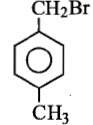(b)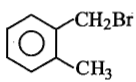(c)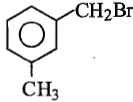(d)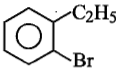Complete Question Bank + Test Series
Complete Question Bank

Difficulty Level:

Which of the following will give yellow precipitate with I2/NaOH?

1. CH3-CO-O-CO-CH3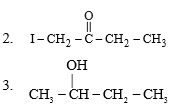4.Both (2) and (3)

Complete Question Bank + Test Series
Complete Question Bank

Difficulty Level:

A nitrogeneous substance X is treated with red P/I2,AgNO2, HNO2 and the product so formed is further treated with NaOH solution, which produces blue colouration. X can be

1. CH3CH2NH2

2 CH3CH2NO2

3. CH3CH2ONO

4 (CH3)2CHNO2

Complete Question Bank + Test Series
Complete Question Bank

Difficulty Level:

The compound which reacts fastest with Lucas reagent at room temperature is:
1. Butan-1-ol
2. Butan-2-ol
3. 2-methylpropan-1-ol
4. 2-methylpropan-2-ol

Complete Question Bank + Test Series
Complete Question Bank

Difficulty Level:

Methanol and ethanol can be distinguished by the following:
1. By reaction with metallic sodium
2. By reaction with caustic soda
3. By heating with iodine and washing soda
4. By heating with zinc and inorganic mineral acid

Complete Question Bank + Test Series
Complete Question Bank

Difficulty Level:

Identify (X) in the sequence :

(1) CH3-CH2-CH2OH

(2)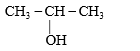(3) CH3-O-CH2-CH3

(4) CH3-CH2-CHO

Complete Question Bank + Test Series
Complete Question Bank

Difficulty Level:

In order to accomplish the following conversion, what reagent and conditions would be required?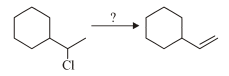1. Cold sodium hydroxide

2. Hot conc. sodium hydroxide

3. Potassium tertiary butoxide and heat

4. Hot water